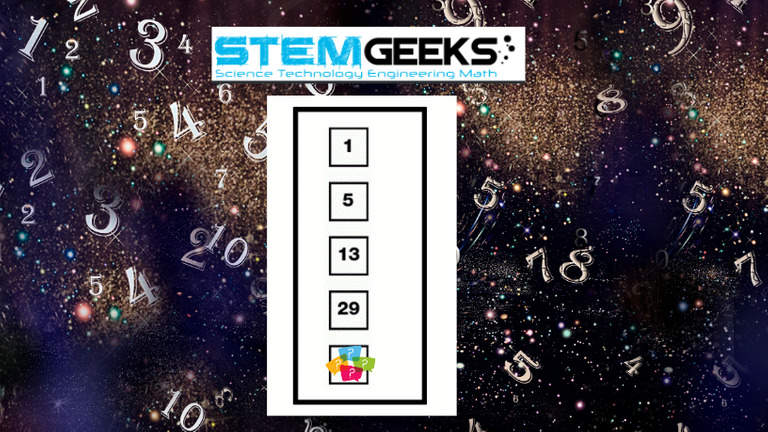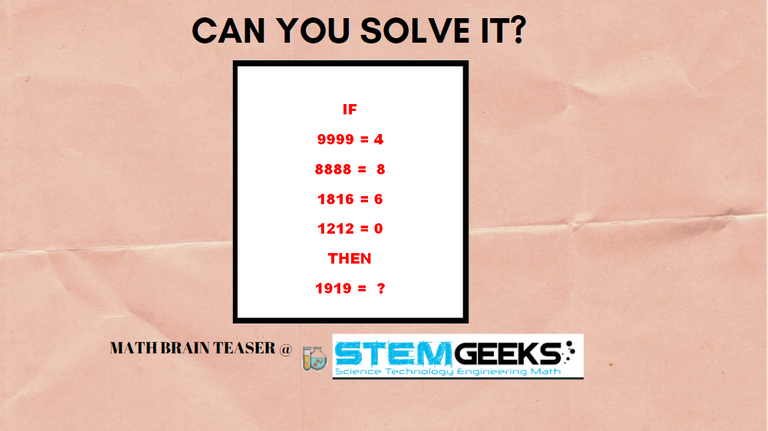# Math Brain Teasers 32:: Can You SOLVE the Sequence Pattern?

in STEMGeeks2 months ago

Hey All; @StemGeeks Mathematician;

Let's start our week by solving this sequence pattern. Following is the Math Brain Teasers for today:: Can You SOLVE the Sequence Pattern?What I'm observing is that when the Maths puzzles get a bit complicated we lose track of solving/trying to solve the puzzles. Anyways this should be an easy sequence pattern to SOLVE for sure. Have a close look at the image and recognize the pattern hidden. With respect to HINT; I would say that its a SIMPLE logic that needs to be applied to get to the answer and replace the question mark with your ANswer...

##### Math Brain Teasers 31:: Can You SOLVE the Logic?- Solved with ExplanationThe logic was to look at how many closed areas there are in an equation i.e. Here is the Question;;

### 1212 has 0 closed areas, (0 * 4 = 0)

Hence the Answer is;

### STEM token GiveAway

I'll be again doing a giveaway of STEM tokens to the lucky random winner with the correct answer. For the last contest, which was Math Brain Teasers 31:: Can You SOLVE the Logic???.

Surprisingly there was no entry to the contest; Math Brain Teasers 31:: Can You SOLVE the Logic?. I thought that this would have been an easy one to solve. . Looks like geeks don't see the puzzle seriously and were not able to solve it. Anyways; I'll see if I can rollover the reward of this contest for the other Maths Brain Teasers contents lined up.

### Math Quote for the Day::

Here is the motivation to solve the Maths Equation/Sequation Puzzle?If you like my work, then please spread the Word.. that we do have the Math Brain Teasers competition here @StemGeeks platform. Reblog is much appreciated.

Best RegardsFind Me on the Other Social Media Platforms::PS:: All the Maths Brain Teasers; are made by me using the Pro Canva License Version

Sort:

61

The next number is 61 :-)

I will also go with 61 :)

The first differences is a pattern as well. It goes up by 4, 8, 16, 32, .. The next number is 61.

That's actually the way I did it. I didn't even look for another method until he posted the answer!